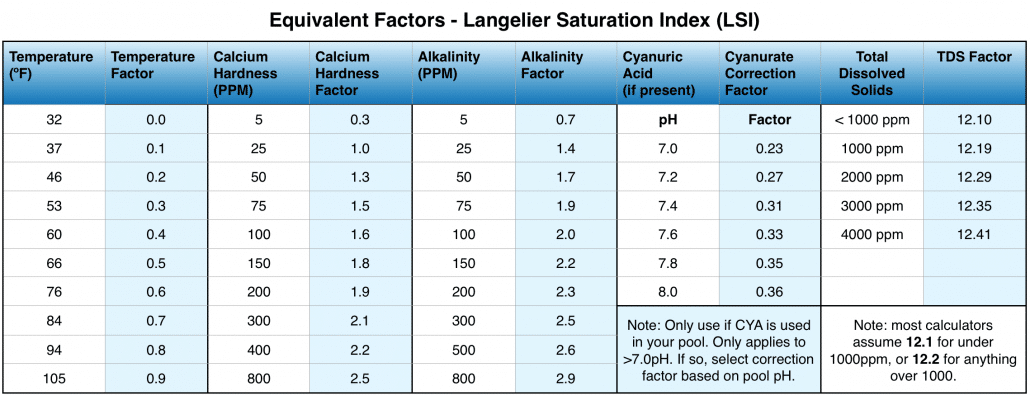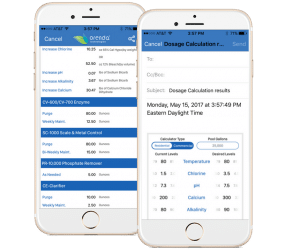You are here: Home / Blog / Understanding the LSI: Langelier Saturation Index

2:03

2:03Thanks for reporting a problem. We'll attach technical data about this session to help us figure out the issue. Which of these best describes the problem?

Any other details or context?

message

The Langelier Saturation Index (LSI) is a formula developed from studies conducted by Dr. Wilfred Langelier in the early 20th century. The LSI is the basis for water balance and saturation, and this article will try to explain how it works in a simplified way. This is complex science, but very helpful to know as a pool owner or operator.

# Think of the LSI as a scale with a fulcrum

A perfect score on the LSI is zero (0.0). Zero is perfectly balanced water; saturated with the perfect amount of calcium and dissolved solids, and has a stable pH. Being the universal solvent, if water is out of balance, it will naturally try to find its own balance and equilibrium, because it wants to be at 0.0 LSI. For instance, if there is not enough calcium, water will dissolve and extract it from the plaster walls of the pool (etching).Calcium carbonate, metals and other dissolved solids must all be saturated and balanced to attain zero LSI.

The Langelier saturation index is basically a way to determine if water is corrosive (negative LSI) or scale-forming (positive LSI). LSI between -0.3 and +0.3 is the widely accepted range, though 0.0 is the ideal.

The key word here is saturation. Ideal saturation is 0.00 LSI. Water wants to be in equilibrium, and will find a way to get there. Under-saturation is corrosive, and over-saturation is scale-forming. Water can only hold so much calcium in suspension. If water is in equilibrium, neither etching nor scaling will happen. As pool professionals, our goal is to balance water up front (and maintain it) to LSI standards so that neither etching nor scaling occur.

Low LSI does not just etch plaster, it can corrode pool equipment too and plastics too.  Yes, that includes vinyl liners and PVC pipe. This is part of the reason why metal sequests are so important to use in pools, especially during start up. Like calcium, water will dissolve metals and keep them in suspension...at least until the water is oversaturated. At that point, metals (most commonly copper, aluminum or magnesium from common pool products such as algaecides) will fall out of solution and can stain pool surfaces.

# How to calculate LSI: it is an equation with six variables

The six variables you need to calculate the Langelier Saturation Index:

• pH
• Temperature (ºF)
• Calcium Hardness (ppm)
• Alkalinity (ppm)
• Cyanuric Acid/Stabilizer (If applicable, correction based on pH)
• Total Dissolved Solids (ppm)

These variables are given numerical equivalent “factors”, as assigned in The Langlier Numerical Equivalents Table. See the chart below.## The LSI Equation

(pH) + (Temperature ºF) + (Calcium Hardness) + [(Total Alkalinity) - (CYA correction factor @ current pH)] - (TDS factor) = LSI

Let’s use an example.

You manage a pool that has the following chemistry:

• ph: (7.4)
• temperature: 84ºF (0.7)
• calcium hardness: 300 (2.1)
• alkalinity: 100 (2.0)
• cyanuric acid: 100 (pH 7.4 = 0.31)
• total dissolved solids < 1000 (12.1)

Let’s calculate.

[(7.4) + (0.7) + (2.1) + [(2.0)-(0.31)] - (12.1) = X LSI

[(10.2) + (1.69)] - (12.1) = X LSI

[11.89] - (12.1) = -0.21 LSI

This is well balanced water (within acceptable range), but errs to the side of corrosive. Ideally, this water could be balanced by increasing the factors in the equation by 0.21. For example, an operator can increase the calcium hardness from 300 to 400, which would add 0.1...which gets closer to perfect balance. Play with the numbers on your own to find the balance that works for you and your pool(s).

See the video on how to use the Orenda LSI Calculator app at the end of this article for more.

# What you need to know about the LSI factors

pH: This variable is the most likely to shift up and down, as pool operators already know. In the LSI calculation, the pH value itself (example: 7.2) is the factor. There is no multiplier. The lower the pH, the more acidic, and the higher the pH, the more alkaline.

Temperature (ºF): Temperature affects the speed of chemical reactions in water. The lower the temperature, the easier it is for corrosive reactions to occur. The higher the temperature, the easier it is for calcium to come out of suspension. This explains why salt cells often have calcium carbonate scale on them (heat).

Calcium Hardness: This is a measurement of how much calcium is dissolved in the water. Water that is over-saturated with calcium is likely to be more scale forming, but only if the pH and total alkalinity allow for it to come out of solution. Calcium hardness—like total alkalinity—serves as a buffer for pH.

Alkalinity: In the original Langelier Saturation Index formula, Dr. Langelier used total alkalinity. Over time, however, it became evident that swimming pool chemistry is different from other types of water; therefore it is more accurate to use carbonate alkalinity. One reason for this change is because many pools use cyanuric acid as a stabilizer.

Because of this, alkalinity requires a mathematical correction when CYA is involved. You can find this in the chart above. Another reason the LSI calls for carbonate alkalinity instead of total alkalinity is because scale is calcium carbonate. So when looking at which type of alkalinity (carbonate, bicarbonate or hydroxide) to measure, carbonate alkalinity is most directly correlated to the formation of scale. Learn more about carbonate alkalinity here.

Cyanuric Acid (stabilizer): If CYA is present, adjust total alkalinity with the cyanurate correction factor in the chart above to find the carbonate alkalinity factor. The CYA correction factor is about 1/3, if you're rounding. To find it exactly, you need to know the pH of the water and follow the chart.

Total Dissolved Solids (TDS): TDS is a measurement of everything that is dissolved in the water, floating around in suspension. It is measured in parts-per-million, and can include anything from salt, to calcium, to metals and other chemicals. Most often, LSI calculations assume either less than 1000ppm or more. But if you want to be exact, use the Orenda App's LSI calculator.

# Things the Langelier Saturation Index teaches us

If you look at the schedule of values, you will see that calcium hardness and alkalinity have a similar impact on LSI. This is important, because it means total alkalinity is not alone in its ability to buffer/stabilize pH. Some experts contend that calcium chloride itself does not buffer pH like alkalinity...and we have no evidence that says it does. The evidence says that various forms of calcium present in your water can buffer pH. (Hint: they're alkaline...)

Anyway, the higher the calcium hardness, the more stable the pH.

Additionally, the LSI offers a different way of looking at water chemistry. By understanding how saturated the water is with metals and calcium, it is a strong predictor of damage to gunite/plaster before it gets bad. And on the other hand, if the LSI is positive, the water is likely to form scale, even if not seen. So as owners and operators, the LSI offers a broader view of water chemistry beyond just pH and chlorine levels. At Orenda, we believe two measurements—LSI and ORP—will be the future standard of measurement in pool chemistry.

## Free LSI calculator available on the Orenda App.2:17

2:17Thanks for reporting a problem. We'll attach technical data about this session to help us figure out the issue. Which of these best describes the problem?

Any other details or context?

message